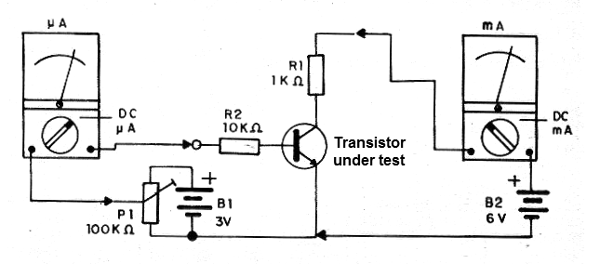### DatasheetsN° of component

## Measuring the Gain of a Transistor (INS035E)

The static (beta) current gain of a transistor can be measured using a simple circuit. For it, we need the following material.

Instruments:

a) Microammeter (multimeter 1)

b) Milliammeter (multimeter 2)

R1 - 1 k ohm x 1/8 W - resistor

R2 - 100 k ohm x 1/8 W - resistor

P1 - 100 k ohm - potentiometer

B1 - 3 V - 2 small batteries

B2 - 6 V - 4 small batteries

Procedure

In the figure below, we have the arrangement to measure the gain of a transistor.To use with PNP transistors, simply reverse the polarity of the batteries.

The procedure is simple. We set P1 until the collector current stops increasing (saturation), or stabilizes at 1 mA (many manuals specify the current gains of the transistors for a current of this magnitude).

We then read the corresponding base current on the second instrument (micro-amps). The relationship between the two currents gives us the static current gain of the transistor.

For example, for a base current of 10 uA and a collector current of 1 mA, the gain will be 100.